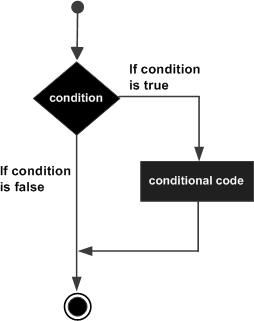# Perl IF Statement

A Perl if statement consists of a boolean expression followed by one or more statements.

## Syntax

The syntax of an if statement in Perl programming language is −

```if(boolean_expression) {
# statement(s) will execute if the given condition is true
}
```

If the boolean expression evaluates to true then the block of code inside the if statement will be executed. If boolean expression evaluates to false then the first set of code after the end of the if statement (after the closing curly brace) will be executed.

The number 0, the strings '0' and "" , the empty list () , and undef are all false in a boolean context and all other values are true. Negation of a true value by ! or not returns a special false value.

## Flow Diagram## Example

```#!/usr/local/bin/perl

\$a = 10;
# check the boolean condition using if statement
if( \$a < 20 ) {
# if condition is true then print the following
printf "a is less than 20\n";
}
print "value of a is : \$a\n";

\$a = "";
# check the boolean condition using if statement
if( \$a ) {
# if condition is true then print the following
printf "a has a true value\n";
}
print "value of a is : \$a\n";
```

First IF statement makes use of less than operator (<), which compares two operands and if first operand is less than the second one then it returns true otherwise it returns false. So when the above code is executed, it produces the following result −

```a is less than 20
value of a is : 10
value of a is :
```
perl_conditions.htm